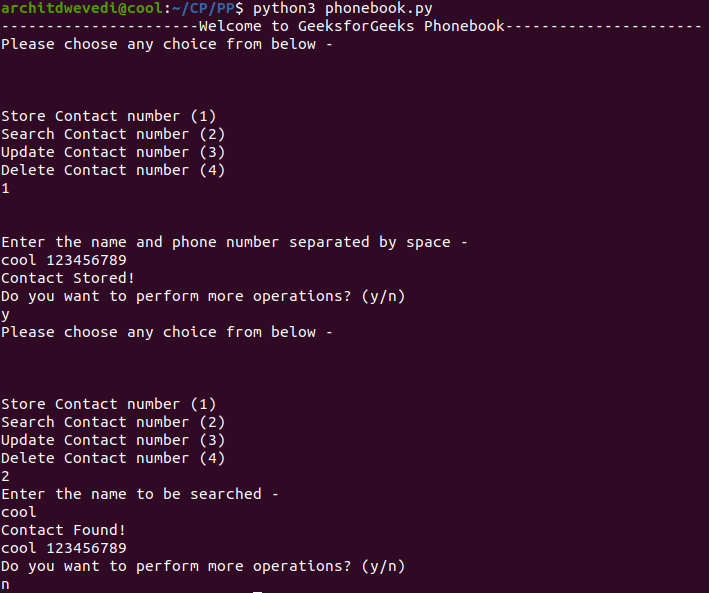Related Articles
Menu-Driven program for Phone Directory
• Last Updated : 17 Jul, 2020

Problem Statement: Write a menu-driven program for using switch-case with following features:

• Store Contact numbers of people
• Search for the Contact numbers using their names
• Search for the Contact numbers using their number
• Delete a Contact number
• Update a Contact number

Examples:

Approach: The idea is to use switch case for switching the case for menu-driven program for the phonebook directory and store the contact number in the hash-map and search the contact in the hash-map in O(1) time.

Below is the implementation of the above approach:

## Python3

 `# Python implementation of menu driven``# Phone Book Directory`` ` `contact ``=` `{}`` ` `# List of Inputs``inputLis ``=` `[``"1"``, ``"cool 123456789"``, ``            ``"y"``, ``"2"``, ``"cool"``, ``"n"``]``indi ``=` `-``1`` ` ` ` `# Function to provide sample inputs``# Remove this function to run on ``# Custom Inputs``def` `input``():``    ``global` `indi``    ``indi ``+``=` `1``    ``print``(inputLis[indi])``    ``return` `inputLis[indi]`` ` `# Function to delete a contact``def` `delete():``    ``global` `contact``    ``print``(``"Enter the contact"``\``        ``" name to be deleted"``)`` ` `    ``name ``=` `input``().strip()`` ` `    ``if` `name ``in` `contact:``        ``del``(contact[name])``        ``print``(``"Contact Deleted !\n"``)``    ``else``:``        ``print``(``"Contact not found !\n"``)`` ` `    ``print``(``"Do you want to perform more"``\``        ``" operations? (y / n)"``)`` ` `    ``choice ``=` `input``().strip()``    ``if` `choice ``=``=` `"y"``:``        ``main()`` ` `# Function to update a contact number``def` `update():``    ``global` `contact``    ``print``(``"Enter the contact name"``\``        ``" to be updated - "``)`` ` `    ``name ``=` `input``().strip()`` ` `    ``if` `name ``in` `contact:``        ``print``(``"Enter the new"``\``            ``" contact number - "``)``        ``phone ``=` `int``(``input``())`` ` `        ``contact[name] ``=` `phone`` ` `        ``print``(``"Contact updated\n"``)``    ``else``:``        ``print``(``"Contact not found !\n"``)`` ` `    ``print``(``"Do you want to perform "``\``        ``"more operations? (y / n)"``)`` ` `    ``choice ``=` `input``().strip()``    ``if` `choice ``=``=` `"y"``:``        ``main()`` ` `# Function to search a contact ``def` `search():``    ``global` `contact``    ``print``(``"Enter the name to be searched - "``)`` ` `    ``name ``=` `input``().strip()`` ` `    ``if` `name ``in` `contact:``        ``print``(``"Contact Found !"``)``        ``print``(name, contact[name])``    ``else``:``        ``print``(``"Contact not found !\n"``)`` ` ` ` `    ``print``(``"Do you want to perform more"``\``        ``" operations? (y / n)"``)`` ` `    ``choice ``=` `input``().strip()``    ``if` `choice ``=``=` `"y"``:``        ``main()`` ` `# Function to store a contact``def` `store():``    ``print``(``"\n\nEnter the name"``\``        ``" and phone number"``+``\``        ``" separated by space - "``)`` ` `    ``name, phone ``=` `map``(``str``, \``                    ``input``().strip()\``                            ``.split(``" "``))`` ` `    ``global` `contact``    ``if` `name ``in` `contact:``        ``print``(``"Contact Already exists !\n"``)``    ``else``:``        ``contact[name] ``=` `phone``        ``print``(``"Contact Stored !"``)`` ` `    ``print``(``"Do you want to perform more"``\``        ``" operations? (y / n)"``)`` ` `    ``choice ``=` `input``().strip()``    ``if` `choice ``=``=` `"y"``:``        ``main()`` ` `# Main Function for Menu-Driven``def` `main():``    ``print``(``"Please choose any choice"``\``        ``" from below -\n\n\n"``)``    ``print``(``"Store Contact number (1)"``)``    ``print``(``"Search Contact number (2)"``)``    ``print``(``"Update Contact number (3)"``)``    ``print``(``"Delete Contact number (4)"``)`` ` `    ``choice ``=` `int``(``input``())`` ` `    ``choice_dict ``=` `{``        ``1``: store,``        ``2``: search,``        ``3``: update,``        ``4``: delete``    ``}`` ` `    ``choice_dict[choice]()`` ` ` ` `if` `__name__ ``=``=` `"__main__"``:``    ``print``(``"----------------------"``+``\``        ``"Welcome to GeeksforGeeks Phonebook"``+``\``        ``"----------------------"``)`` ` `main()`

Output:Attention reader! Don’t stop learning now. Get hold of all the important DSA concepts with the DSA Self Paced Course at a student-friendly price and become industry ready.

My Personal Notes arrow_drop_up